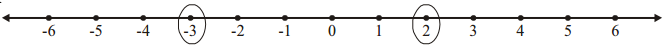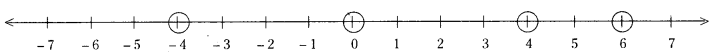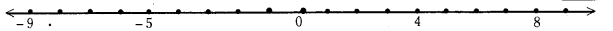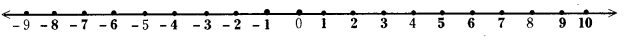# AP Board 7th Class Maths Solutions Chapter 1 Integers Ex 1

AP State Syllabus AP Board 7th Class Maths Solutions Chapter 1 Integers Ex 1 Textbook Questions and Answers.

## AP State Syllabus 7th Class Maths Solutions 1st Lesson Integers Exercise 1Question 1.
Some integers are marked on the number line. Which is the biggest and which is the
smallest?Solution:
The numbers marked = -3 and 2
biggest number = 2
smallest number = -3

Question 2.
Write the integers between the pairs of integers given below. Also, choose the biggest and
smallest intergers from them.
(i) – 5, – 10
(ii) 3, – 2
(iii) – 8, 5
Solution:
i) – 5, – 10
Integers between – 5, – 10 are – 6, – 7, – 8, – 9
smallest Integer – 9
biggest integer = – 6

ii) 3, – 2
Integers between 3 and – 2 are – 1,0, 1, 2
smallest integer = – 1
biggest integer 2

iii) – 8, 5
Integers between – Sand 5 are 4, 3, 2, 1, 0, – 1, – 2, – 3, – 4, – 5, – 6, – 7
smallest integer = – 7
biggest integer = 4Question 3.
Write the following integers in ascending order (smallest to biggest).
(i) – 5, 2, 1, – 8
(ii) – 4, – 3, – 5, 2
(iii) – 10, – 15, – 7
Solution:
(i) – 8. – 5, 1, 2
(ii) – 5, – 4, – 3, 2
(iii) – 15, – 10, – 7

Question 4.
Write the following integers in descending order (biggest to smallest).
(i) – 2, – 3, – 5
(ii) – 8, – 2, – 1
(iii) 5, 8, – 2
Solution:
(i) – 2, – 3, – 5
(ii) – 1, – 2, – 8
(iii) 8, 5, – 2Question 5.
Represent 6, – 4, 0 and 4 on a number line.
Solution:Question 6.
Fill the missing integers on the number line given belowSolution:Question 7.
The temperatures of 5 cities in india on a particular day are shown on the number line below.(i) Write the temperatures of the cities marked on it?
(ii) Which city has the highest temperature?
(iii) Which city has the lowest temperature?
(iv) Which cities have temperature less than 0°C?
(v) Name the cities with temperature more than 0°C?
Solution:
i) The temperatures of the cities shown on the nL.lber line an
Kasauli
Manali = – 7°C
Nainital = – 3°C
Ooty = 15°C
l3anglore = 20°C

ii) The city with highest temperature is Banglore.
iii) The city with lowest temperature is Kasauli.
iv) The cities with temperatures less than 0°C are Nainital, Manali and Kasauli.
v) The cities with temperatures more than 0°C are Ooty and Banglore.